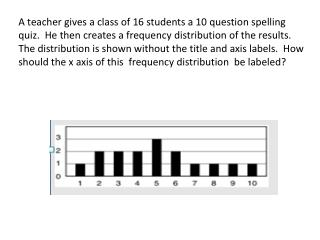DownloadDownload PresentationCartesian Plane

# Cartesian Plane

Download Presentation## Cartesian Plane

- - - - - - - - - - - - - - - - - - - - - - - - - - - E N D - - - - - - - - - - - - - - - - - - - - - - - - - - -
##### Presentation Transcript

1. A teacher gives a class of 16 students a 10 question spelling quiz. He then creates a frequency distribution of the results. The distribution is shown without the title and axis labels. How should the x axis of this frequency distribution be labeled?

3. The development of the Coordinate or Cartesian plane is often credited to the French philosopher and mathematician, Rene Descartes. It is said that Descartes first came up with the idea for the plane as he lay in bed watching several flies crawl across his tiled ceiling; as he observed their movement he realized that he could use the intersecting lines formed by the tiles to describe a fly’s location. Although historical evidence suggests that a contemporary of Descartes, Pierre de Fermat, did more to develop the coordinate system, Rene Descartes’ work certainly revolutionized mathematics by describing the properties of the plane and using it as the first systematic link between Euclidean geometry and algebra. " " Cogito,ergo sum Pierre, bring me my fly swatter! Rene Descartes 1596 - 1650 (1,1) x The well known quote; "Cogito,ergo sum" (I think,therefore I am) is attributed to Rene Descartes. (-1,-1) (2,-2) y Fun Fact

4. 0 The coordinate plane is divided into four sections called quadrants. Each quadrant is numbered using the Roman numerals I through IV, in a counter-clock wise direction.

5. C Slide the "C" onto the coordinate plane 0 The Coordinate plane is also called the Cartesian plane. One way to remember how the quadrants are numbered is to write a big "C" on top of the plane. The "C" will begin in quadrant I and end in quadrant IV.

6. y - axis 0 x - axis The quadrants are formed by two intersecting number lines called axes. The horizontal line is the x-axis. The vertical line is the y-axis.

7. Origin (0, 0) 0 The point at which the x and y axes intersect is called the origin. The coordinates of the origin are (0, 0).

8. 0 Points can be plotted on the plane using one coordinate from each of the axes. These sets are called ordered pairs. The x coordinate always appears first in these pairs. The y coordinate appears second. (x, y)

9. Each of the quadrants can be identified by the properties of the numbers that fall within their plane. Remember the ordered pairs are always of the form (x, y) (-,+) ( +,+) 0 (+,-) (-,-)

10. 56 What points are in quadrant II ? A B C D E F

11. 57 What points are in quadrant I ? A B C D E F

12. To graph an ordered pair, such as(3,2): • start at the origin (0,0) • moveleft or right on the x-axisdepending on thefirst number • then moveup or downfrom there depending on thesecond number • plot the point

13. Write what to do. To graph (-3,4): Start at the origin and then move 3 left, up 4

14. To graph(-3,-2): Start at the origin and then move 3 left, down 2

15. To graph(5,-3): Start at the origin and then move 5 right, down 3

16. Place the star on (2,8) in  quadrant I   Place the triangle on (-4, 4) in quadrant II Place the square on (-7, -3) in quadrant III Place the circle on (1, -4) in quadrant IV

17. Place the circle on (-7,-5) Place the star on (4,9) Place the triangle on (-6,2) Place the square on (3,-9) In which quadrant is the circle?